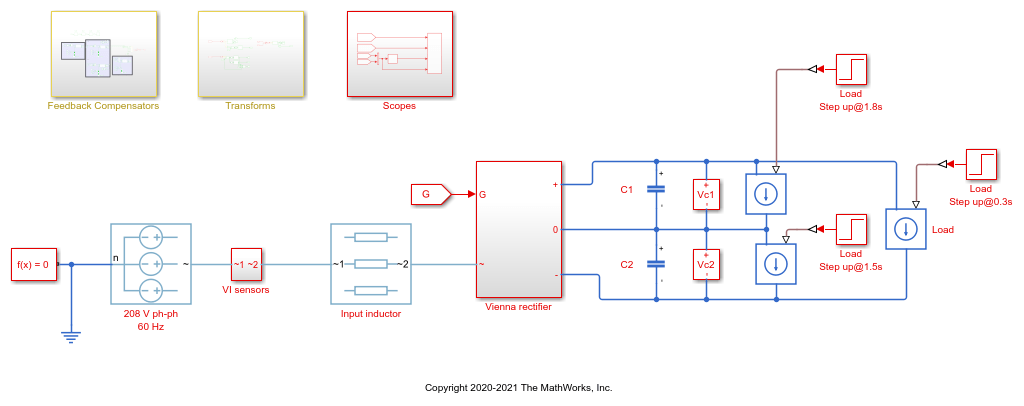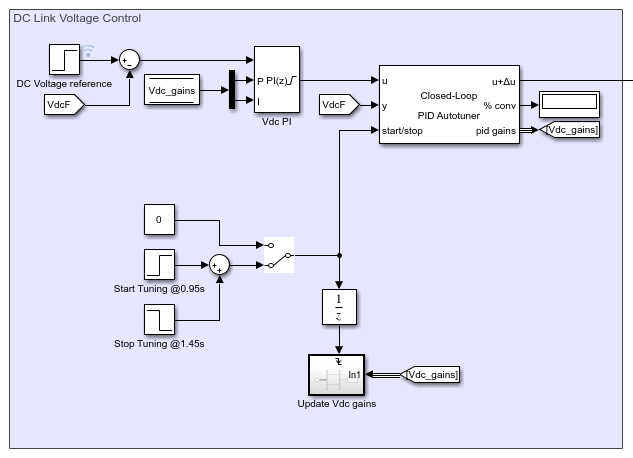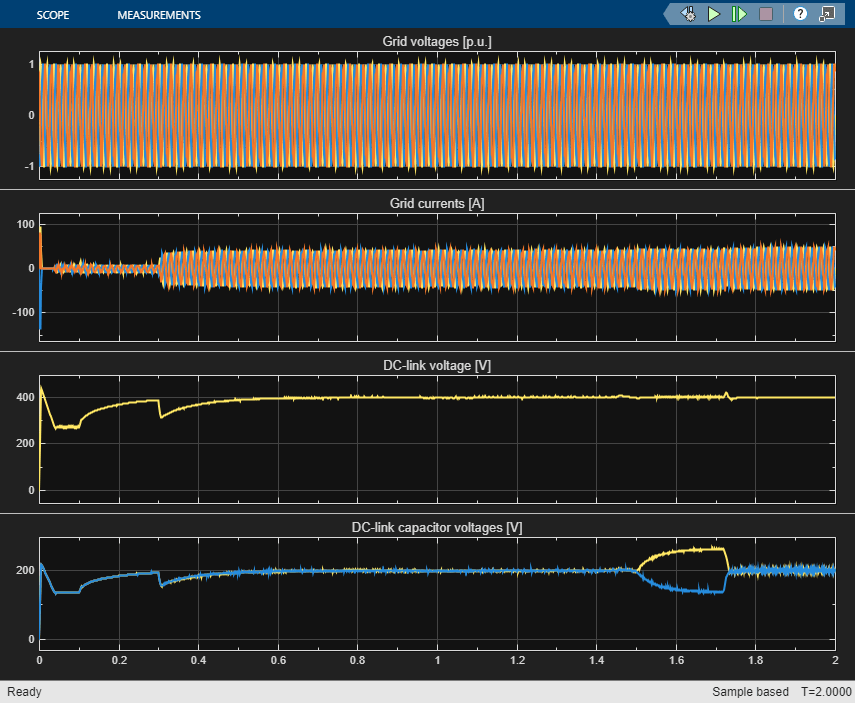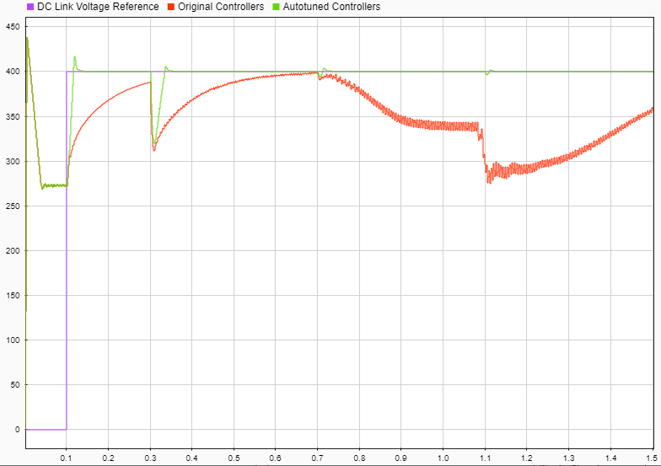Main Content

# Design PID Controllers for Three-Phase Rectifier using Closed-Loop PID Autotuner Block

This example shows how to use the Closed-Loop PID Autotuner block to tune the DC-link voltage, DQ axis current, and voltage neutral controllers for a Vienna-rectifier-based power factor corrector.

### Power Factor Correction Model

This example uses the power factor correction circuit described in Vienna Rectifier Control (Simscape Electrical). Power factor correction preconverters correct the power factor of loads, which increases the energy efficiency of the distribution system. This correction is useful when nonlinear impedances, such as switched-mode power supplies, are connected to the AC grid.

This model uses a Vienna rectifier and a switched-mode power supply to convert a three-phase 120V AC supply to a regulated 400V DC supply. To ensure that the device on-resistances are correctly represented, the semiconductor components are modeled using MOSFETs rather than ideal switches. The model simulation is configured to run in accelerator mode using the partitioning solver.

```open_system('PWM_Rectifier_Vienna_SC') ```### DQ-Axis Current Control

For this example, the DQ-axis controller for the Vienna rectifier is modeled as shown in the following diagram.In DQ-axis control, the time-dependent, three-phase currents are transformed into a time-invariant, two-coordinate vector using projections. These transformations are the Clarke Transformation, the Park Transformation, and their respective inverse transformations. These transformations are implemented as blocks within the Measurements subsystem. To maintain a power factor close to 1, the reactive power being drawn from the grid should be close to zero. Therefore, commanding a zero Q-axis current from the controller allows the power factor to be close to 1.

In the model, the controllers have the following gains:

• DC-link voltage PI controller: P = 2 and I = 20

• Both DQ-axis current PI controllers: P = 5 and I = 500

• Voltage neutral P controller: P = 0.001

The controller gains are stored in a Data Store Memory block and provided externally to each PID block. When the tuning process for a controller is complete, the new tuned gains are written to the Data Store Memory block. This configuration allows you to update your controller gains in real-time during the simulation.

For this example, you retune these controllers using Closed-Loop PID Autotuner blocks.

### Closed-Loop PID Autotuner Block

The Closed-Loop PID Autotuner block allows you to tune one PID controller at a time. It injects sinusoidal perturbation signals at the plant input and measures the resulting plant output during a closed-loop experiment. When the experiment stops, the block computes PID gains based on the plant frequency responses estimated at a small number of points near the desired bandwidth. For this Vienna rectifier model, the Closed-Loop PID Autotuner block can be used for each of the controllers, as shown for the DC link voltage loop below.This workflow applies when you have initial controllers that you want to retune using the Closed-Loop PID Autotuner block. The benefits of this approach are:

1. If there is an unexpected disturbance during the experiment, it is rejected by the existing controller to ensure safe operation.

2. The existing controller keeps the plant running near its nominal operating point by suppressing the perturbation signals.

When using the Closed-Loop PID Autotuner block for both simulations and real-time applications:

• The plant must be either asymptotically stable (all the poles are strictly stable) or integrating. The autotuner block does not work with an unstable plant.

• The feedback loop with the existing controller must be stable.

• To estimate plant frequency responses more accurately in real time, minimize the occurrence of any disturbance in the Vienna rectifier model during the experiment. The autotuner block expects the plant output to be the response to the injected perturbation signals only.

• Because the feedback loop is closed during the experiment, the existing controller suppresses the injected perturbation signals as well, which reduces the accuracy of frequency response estimation when your target bandwidth is far away from the current bandwidth.

### Tuning Cascaded Feedback Loops

Since the Closed-Loop PID Autotuner block only tunes one PID controller at a time, the four controllers must be tuned separately in the model. Therefore, you tune the inner current controllers first, followed by the DC-link voltage controller, and then the voltage neutral controller.

During the model simulation:

• The D-axis current controller is tuned between 0.65 and 0.75 sec.

• The Q-axis current controller is tuned between 0.8 and 0.9 sec.

• The DC-link voltage controller is tuned between 0.95 and 1.45 sec.

• The voltage neutral controller is tuned between 1.7 and 1.72 sec.

After tuning each of the controllers, the controller gains are updated through the Data Store Memory block.

### Configure Autotuner Block

After connecting the Closed-Loop PID Autotuner blocks with the plant and the PID blocks, configure the tuning and experiment settings for each of them. On the Tuning tab, there are two main tuning settings:

• Target bandwidth - Determines how fast you want the controller to respond. In this example, choose 3000 rad/sec for the current control, 400 rad/s for the DC-link voltage control, and 20000 rad/s for the voltage neutral control.

• Target Phase Margin - Determines how robust you want the controller to be. In this example, choose 60 degrees for all the controllers.

On the Experiment tab, there are three main experiment settings:

• Plant Type - Specifies whether the plant is asymptotically stable or integrating. In this example, the Vienna rectifier model is stable.

• Plant Sign - Specifies whether the plant has a positive or negative sign. The plant sign is positive if a positive change in the plant input at the nominal operating point results in a positive change in the plant output when the plant reaches a new steady state. Otherwise, the plant sign is negative. If a plant is stable, the plant sign is equivalent to the sign of its DC gain. If a plant is integrating, the plant sign is positive (or negative) if the plant output keeps increasing (or decreasing). In this example, the Vienna rectifier model has a positive plant sign.

• Sine Amplitudes - Specifies the amplitudes of the injected sine waves. In this example, to ensure that the plant is properly excited within the saturation limit, choose `0.6` for the D-axis controller, `0.19` for the Q-axis controller, `1` for the DC-link voltage controller, and `0.01` for the voltage neutral controller. If the excitation amplitude is either too large or too small, it will produce inaccurate frequency response estimation results for these experiments.

### Simulate Autotuner Block in Accelerator Mode

In this example, the Vienna rectifier model is run in accelerator mode and all four controllers are tuned in one simulation. The simulation of the model usually takes a few minutes due to the small sample time of the power electronics controller.

To tune the controllers, simulate the model.

```sim('PWM_Rectifier_Vienna_SC') ```
```ans = Simulink.SimulationOutput: logsout: [1x1 Simulink.SimulationData.Dataset] tout: [4000001x1 double] SimulationMetadata: [1x1 Simulink.SimulationMetadata] ErrorMessage: [0x0 char] ```

The graph below shows the DC-link voltage profile during the current and voltage controller tuning from 0.65 to 1.45 seconds. It also shows the introduction of an unbalanced load at 1.5 seconds and the subsequent voltage neutral controller tuning at 1.7 seconds.

```open_system('PWM_Rectifier_Vienna_SC/Scopes/Scope') ```The four controllers are tuned with the new gains.

• DC-link voltage PI controller: P = 0.7386 and I = 135.6

• D-axis current PI controllers: P = 8.407 and I = 1127

• Q-axis current PI controllers: P = 11.91 and I = 3706

• Voltage neutral P controller: P = 6.628

The graph below shows the DC-link voltage response in comparison to the reference before and after tuning the controllers. The original controller (red) is unable to maintain the DC-link voltage after the introduction of unbalanced loads at 0.7 and 1.1 seconds. On the other hand, the autotuned controller decreases the rise time with minimal overshoot and a good settling time to the steady-state value.## SupportGet trial now